SSC BOARD PAPERS IMPORTANT TOPICS COVERED FOR BOARD EXAM 2024

# Laws Of Motion

##### Class 9th Science & Technology MHB Solution
Exercises
1. Match the first column with appropriate entries in the second and third columns and remake…
2. Distance and displacement Clarify the differences
3. Uniform and non-uniform motion Clarify the differences
4. Complete the following table.
5. Complete the sentences and explain them. A. The minimum distance between the start and…
6. When an object falls freely to the ground, its acceleration is uniform. Give scientific…
7. Even though the magnitudes of action force and reaction force are equal and their…
8. It is easier to stop a tennis ball as compared to a cricket ball, when both are travelling…
9. The velocity of an object at rest is considered to be uniform. Give scientific reasons.…
10. Take 5 examples from your surroundings and give explanation based on Newton's laws of…
11. An object moves 18 m in the first 3 s, 22 m in the next 3 s and 14 m in the last 3 s. What…
12. An object of mass 16 kg is moving with an acceleration of 3 m/s^2 . Calculate the applied…
13. A bullet having a mass of 10 g and moving with a speed of 1.5 m/s, penetrates a thick…
14. A person swims 100 m in the first 40 s, 80 m in the next 40 s and 45 m in the last 20 s.…

###### Exercises
Question 1.

Match the first column with appropriate entries in the second and third columns and remake the table.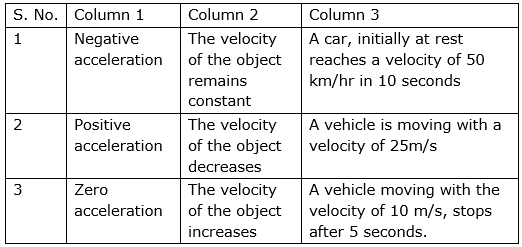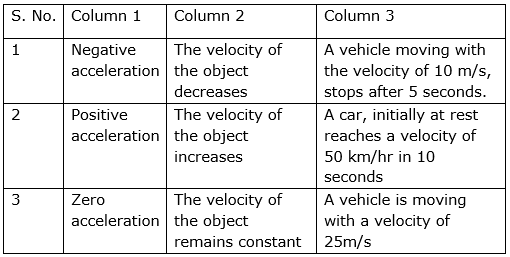The velocity-time graphs are given below: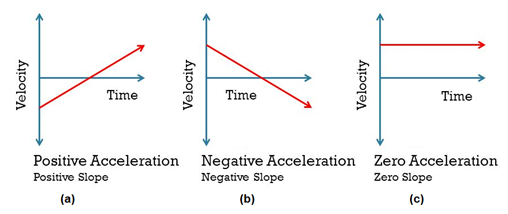Question 2.

Clarify the differences

Distance and displacement

i. Distance refers to "how much length an object has covered" during its motion. And, displacement refers to "how far out of place an object is"; It is the object's overall change in position.

ii. It depends only on magnitude not on direction. Displacement depends on both magnitude as well as direction.

iii. The diagram is given below: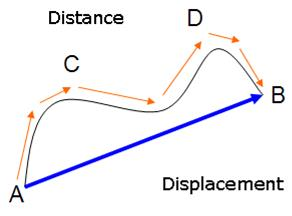In the above diagram,

AB is the distance

ACDB is the distance travelled by the object.

iv) Distance is a scalar quantity as it has only magnitude whereas Displacement is a vector quantity as it has both magnitude and displacement.

Question 3.

Clarify the differences

Uniform and non-uniform motion

(i) The difference between uniform and non-uniform motion relies on, whether the velocity of the moving body is changing or not. If the velocity of the object changes to particular rate, then the motion is uniform, but if it increases or decreases at different points of time, then this movement is called as non-uniform motion.

(ii) In uniform motion, the object covers equal distance in equal time interval. In non-uniform motion, object covers equal distances in unequal time-intervals.

(iii)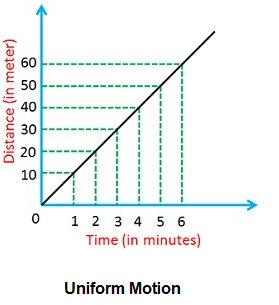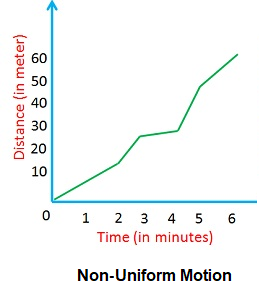Question 4.

Complete the following table.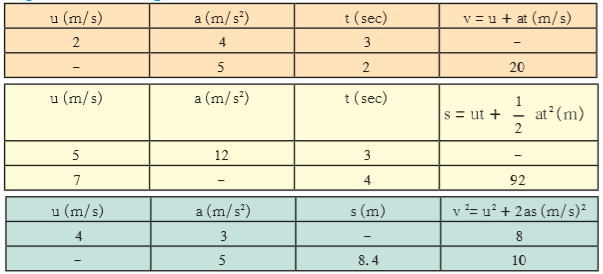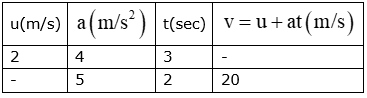From the first equation of motion,

v=u+at

here,

v = final velocity

u = initial velocity

a is the acceleration

t is the time

In the first table,

From Ist row, v = ?

⇒ v =2m/s + 4ms-2 × 3s

⇒ v = 2m/s + 12 m/s

⇒ v = 14 m/s

from 2nd row, u = ?

v = u + at

⇒ 20 m/s = u + 5ms-2 × 2s

⇒ 20 m/s – 10m/s = u

⇒ u = 10 m/s

Now,

In the second table,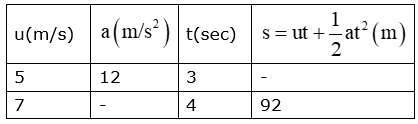From the equation of motion,

S=ut + 1/2 at2

Where, s is the distance

“u” is the initial velocity.

“v” is the final velocity.

“t” is the time.

“a” is the acceleration

From Ist row, s = ?

⇒ S=ut + 1/2 at2

⇒ S = 5ms-1 × 3s + 1/2 × 12ms-2 × 3s × 3s

⇒ S=15m+ 54m

⇒ S = 69 m

From 2nd row, a = ?

⇒ S=ut + 1/2 at2

⇒ 92m = 7ms-1 × 4s + 1/2 × a × 4s ×4s

⇒ 92 m = 28 m + a × 8s2

⇒ a × 8s2 = 92 – 28 m

⇒ a × 8s2 = 64 m

⇒ a= 8 m/s2

Now, for the third table,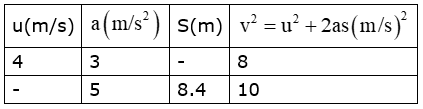From second equation of motion,

v2 = u2+2as

From Ist row ⇒ 8 = 4 × 4 + 2 × 3 × S

⇒ 8 = 16 + 6 × S

⇒ -8 = 6 × S

⇒ -8/6= S

⇒ S= -3/2 =-1.5 m

From 2nd row ⇒ 10= u2+ 2× 5× 8.4

⇒ 10=u2+84

⇒ -74=u2

⇒ u=-8.6m/s

Question 5.

Complete the sentences and explain them.

A. The minimum distance between the start and finish points of the motion of an object is called the ______ of the object.

B. Deceleration is ______ acceleration

C. When an object is in uniform circular motion, its ______changes at every point.

D. During collision ______remains constant.

E. The working of a rocket depends on Newton’s ______ law of motion.

A. The minimum distance between the start and finish points of the motion of an object is called the displacement of the object.

Explanation:

As seen in the given figure,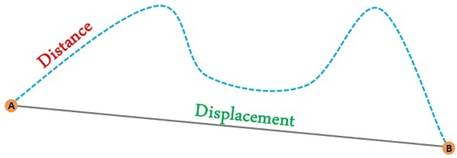The distance is not same as displacement. In fact, the displacement is the shortest distance between two given points.

B. Deceleration is negative acceleration.

Explanation:

Acceleration means increase in velocity. And, for decrease in velocity we have another term called “negative acceleration or deceleration”.

The figure shows the velocity time graph of an object: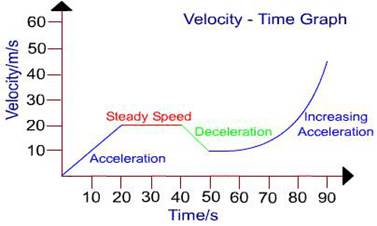C. When an object is in uniform circular motion, its velocity changes at every point.

Explanation:

In uniform circular motion, the speed of the object is same. Since, it direction is changing at every instant, hence its velocity changes. Because, velocity is speed with direction.

The object in uniform circular motion is shown here: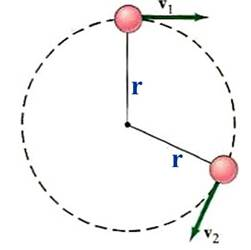Here, the magnitude of both velocities is same.

D. During collision total momentum remains constant.

Explanation:

During collision the total momentum, remains the same.

The figure shows two objects here before & after collision: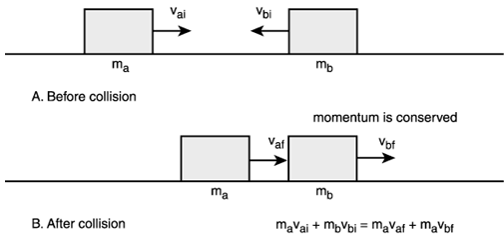E. The working of a rocket depends on Newton’s third law of motion.

Explanation: According to Newton’s third law of motion, every action has an equal and opposite reaction.

In a rocket, the burnt fuel releases gases, which push the rocket upwards. The direction of forces is given in the figure below: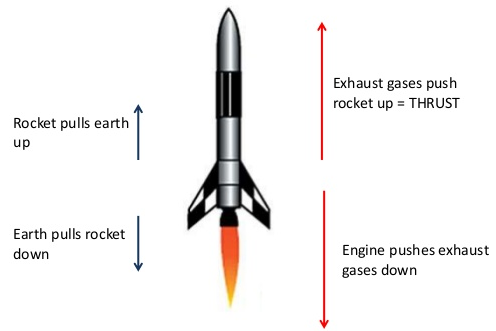Question 6.

Give scientific reasons.

When an object falls freely to the ground, its acceleration is uniform.

As we know thatWhere , G'= new gravitational acceleration

G = gravitational acceleration initial (9.8 m/sec2)
H = Height from the surface of Earth
The height from which the body will be H<<Re
This means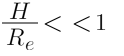We get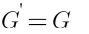Hence , the acceleration of free falling body is uniform on the surface of Earth and Decreases with the height of the body.

Question 7.

Give scientific reasons.

Even though the magnitudes of action force and reaction force are equal and their directions are opposite, their effects do not get cancelled.

i. When the point of action of the two equal and opposite forces is the same, then these do cancel out.

ii. But when the point of action of the two equal and opposite forces is different Though it located on the same body but separated by some distance between a the points of action of the two forces, then these forces don’t cancel out, but rather produce a couple. Example, closing a tap.

iii. But, if they are acting on different bodies, they cannot cancel out but rather produce motion.

For example, the downward weight is cancelled by the normal force while the force of the sun on the earth and its reaction, the force of the earth on the sun, do not cancel each other. We can see from diagram also.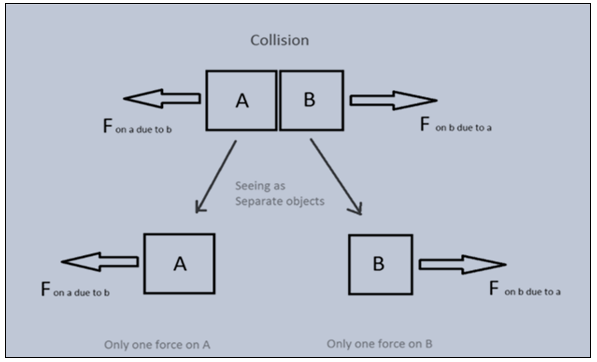Question 8.

Give scientific reasons.

It is easier to stop a tennis ball as compared to a cricket ball, when both are travelling with the same velocity.

Cricket ball is heavier than the tennis ball. Hence, the force required to stop a cricket ball is more as compared to tennis ball with the same velocity.

As we know, Force = Mass × acceleration

Here, force is directly proportional to mass.

So even if the velocity is same, the mass of leather ball is greater than that of tennis ball and the momentum (which is mass multiplied by velocity) and hence has more momentum and it is not easier to stop cricket ball.

Question 9.

Give scientific reasons.

The velocity of an object at rest is considered to be uniform.

In a uniform motion, the object travels equal distances in equal interval of time. Also, in uniform motion, the speed of the object is constant. The graph is given below: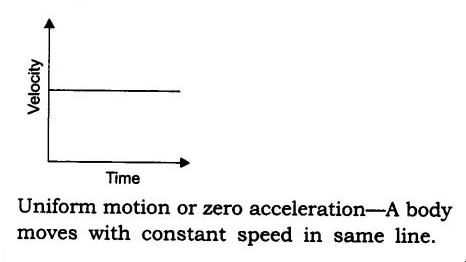Now, for an object at rest, the speed of the object is zero. As the time passes by, the speed is still zero. Hence, the object at rest is also considered as object in uniform motion.

Question 10.

Take 5 examples from your surroundings and give explanation based on Newtons laws of motion.

1) Newton’s law of motion : Explanation with examples

According to the first law: If an object is at rest, it will remain in rest ,in motion will remain in motion unless an external unbalanced force act on it.

Example 1: Pushing a big rock, the rock will stay in place until you push it.

Example 2. When a rocket goes up in space, there is almost a perfect amount of earth gravity for ship to not come crashing down.

Example 3. When we shot the bullet in a space, it would travel in straight line until it reaches gravity of another planet.

2) Newton’s law of motion : Explanation with examples

According to 2nd law: The force is directly proportional to rate of change of momentum.

Example 1: If a truck and bicycle did a short race at the same speed, then bicycle will win the race.

Example 2: For a long race truck will win the race because F=ma and a(acceleration) is more.

Example 3: If a dog is pulling a rock then acceleration is fully dependent on force of dog because mass of rock will not change.

3) Newton’s law of motion : Explanation with examples

According to the 3rd law of motion, for every action there is an equal and opposite reaction.

Example 1. When we push the floor, the floor pushes back.

Example 2. Cars wheel produce friction trying to push and ground exerts an opposite force to push it.

Example 3. Rockets use fuel to push on ground ,which lifts them up. Rockets also rely on friction with air so that the fuel still works.

Question 11.

Solve the following examples.

An object moves 18 m in the first 3 s, 22 m in the next 3 s and 14 m in the last 3 s. What is its average speed?

We know,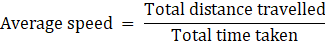⇒ Average speed =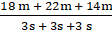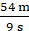= 6m/s

∴ the average speed is 6 m/sec.

Question 12.

Solve the following examples.

An object of mass 16 kg is moving with an acceleration of 3 m/s2. Calculate the applied force. If the same force is applied on an object of mass 24 kg, how much will be the acceleration?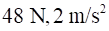F= ma

Where m=16kg

a=3m/s2

F=16× 3= 48 N

If F= 48 N

m= 24kg

F= ma

48=24× a

a= 2 m/s2

Question 13.

Solve the following examples.

A bullet having a mass of 10 g and moving with a speed of 1.5 m/s, penetrates a thick wooden plank of mass 90 g. The plank was initially at rest. The bullet gets embedded in the plank and both move together. Determine their velocity.

Given:

Mass of bullet, m1 = 10g = 0.01 kg

Speed of bullet, v1 = 1.5 m/sec

Mass of wooden plank, m2 = 90 g = 0.09 kg

Velocity of plank + bullet system = ?

Here, we will apply the law of conservation of momentum,

i.e. Final momentum = initial momentum

If we take bullet and thick wooden plank as a system then, initially only bullet is moving with speed of 1.5 m/s and plank is in rest.

∴ initial Momentum (pi) = mass of bullet × velocity

= 0.01 kg ×1.5 m/sec

= 0.015 kgms-1

⇒ pi = 0.015 kgms-1 ….(1)

Now, when the bullet hits the plank, then both the objects are attached.

So, new mass(M) = mass of bullet + mass of plank

= 0.01 kg + 0.09 kg

= 0.1kg

Then,

Final momentum, pf = New mass (M) × (velocity of plank & bullet system)

⇒ pf = 0.1kg × v….(2)

Now, from equation (1) & (2), we get,

Pi = pf

⇒ 0.015 kgms-1 = 0.1kg × v

⇒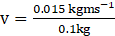⇒ v = 0.15 m/s

Question 14.

Solve the following examples.

A person swims 100 m in the first 40 s, 80 m in the next 40 s and 45 m in the last 20 s. What is the average speed?

2.25 m/s

The average speed is given as: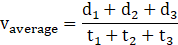⇒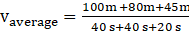⇒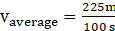⇒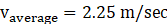Therefore, the average speed is 2.25 m/sec